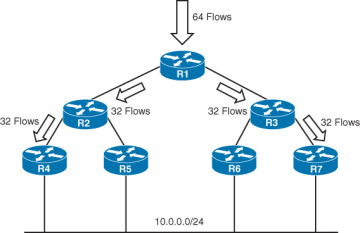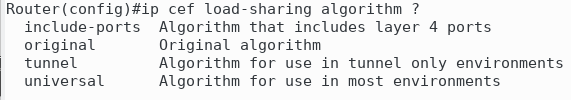Moving on from load-balancing, let’s discuss CEF polarization, which is a problem already solved, however is still important to study.Let’s take the above diagram where all routers are utilizing the older iteration of CEF without Universal IDs.

If 64 flows come into this router, CEF will equally distribute them across its 2 links.

The second hop is where the problem starts, R2 utilizes the same hashing function as R1, thus the hashing result should be the same.
This sends all of the flows across one path, because they all hash the same way. This concept is known as CEF polarization.

We need a bit of entropy introduced into the hashing formula such that one flow does not have the same hash across two different routers.

Cisco introduced a locally significant value, known as the Universal ID to fix this problem. Each router has a random, and different universal ID.
This ID is used as part of the computation of the hash for load balancing in CEF. Thus guaranteeing that hash of each flow will be different per hop.

This leads us to the introduction of different algorithm interations that CEF has gone through:

1. Original algorithm CEF, which was vulnerable to CEF polarization
2. Universal algorithm CEF, which introduced the Universal ID, which was NOT vulnerable to CEF polarization
3. Tunnel algorithm CEF, which was a one off implementation designed to avoid load balancing issues with highly tunnel’d environments. Not vulnerable to CEF polarization.
4. L4 port algorithm CEF, which was based on the universal algorithm CEF, however this introduced L4 source and destination ports to further separate flows. Not vulnerable to CEF polarization.

To change CEF’s load balancing algorithm, utilize the following command:Note when changing this on the Catalyst 6500 series, the commands differ, they utilize “MLS ip cef load-sharing”.